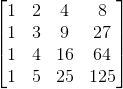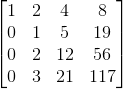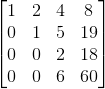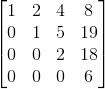# GATE | GATE CS 2019 | Question 53

Consider the following matrix:The absolute value of the product of Eigenvalues of R is _________ .

Note: This was Numerical Type question.
(A) 12
(B) 145
(C) 125
(D) 4

Explanation: The determinant of a matrix is equal to the product of eigenvalues. So, the determinant of above matrix will be calculated as follows:

Apply row operation R2 → R2 – R1 and R3 → R3 – R1 and R4 → R2 – R1Apply row operation R3 → R3 – 2 * R2 and R4 → R4 – 3 * R2Apply row operation R4 → R4 – 3 * R3So, determinant of above matrix is,

```= 6 * (2 * (1*1))
= 12 ```